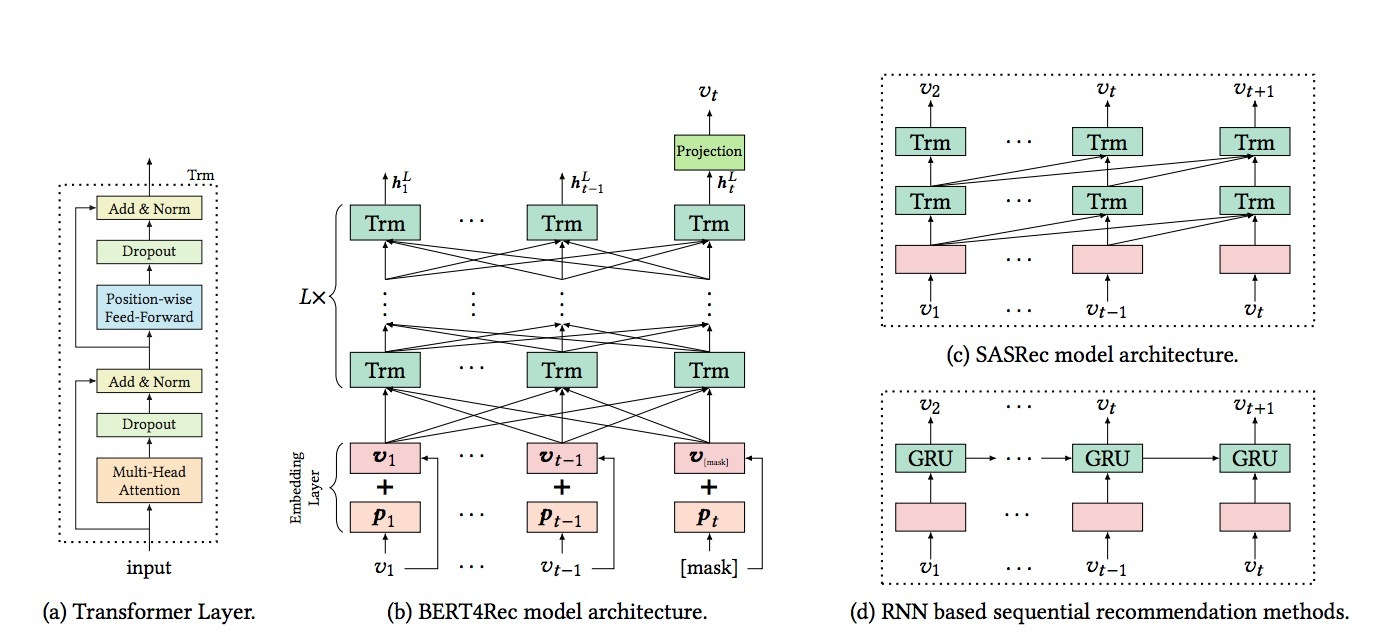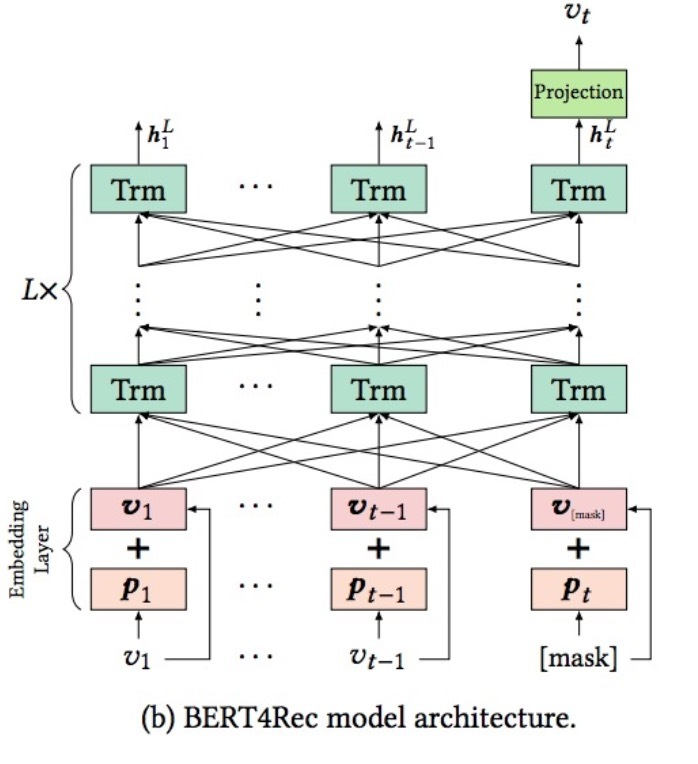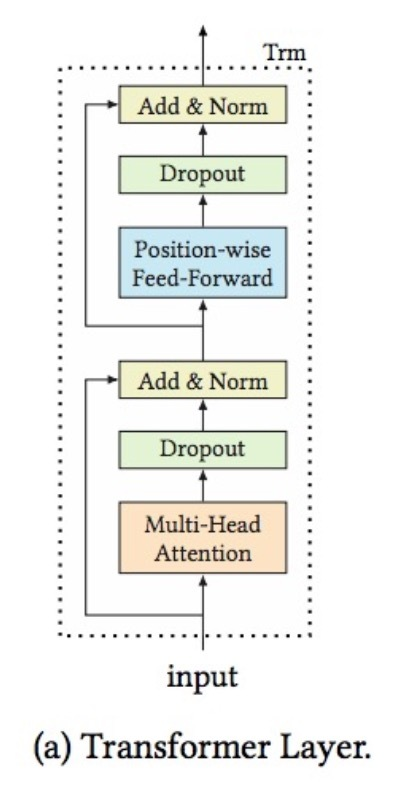youtube在2019发布了它的双塔模型《Sampling-Bias-Corrected Neural Modeling for Large Corpus Item Recommendations》：

# 介绍

• 1) 分别为{user,context}和{item}各自学习query和item representations
• 2) 在query和item representations间使用一个simple scoring function（比如：dot product）来得到对该query合适的推荐

context通常表示具有动态特性的variables，比如：天时长(time of day)，用户所用设备（devices）。representation learning问题通常有以下两个挑战：

• 1) items的corpus对于工业界规模的app来说相当大
• 2) 从用户反馈收集得到的训练数据对于某些items相当稀疏• Streaming Frequency Estimation：我们提出了一个新算法，根据vocabulary和分布偏移（distribution shifts）来估计来自data stream的item frequency。我们提供了分析结果来展示该estimation的variance和bias。我们也提供了仿真来演示我们的方法在捕捉数据动态性上的效率
• Modeling Framework：我们提供了一个通用的建模框架来构建大规模检索系统。特别的，我们针对batch softmax会在cross entropy loss中引入estimated item frequency来减小在in-batch items上的sampling bias

# 2.相关工作

## 2.1 content-aware&Neural Recommenders

• 1) 我们利用一个two-tower NN来建模user-item interactions，以便可以在sub-linear时间内实现在大语料items的inference。
• 2) 学习NCF依赖于point-wise loss（比如：squared或log loss），而我们会引入multi-class softmax loss以及显式的model item frequency。

# 3.模型框架

$u: X \times R^d \rightarrow R^k, v: Y \times R^d \rightarrow R^k$

$s(x,y) = <u(x,\theta), v(y,\theta)>$

$\mathscr{T} := \lbrace (x_i, y_i, R_i) \rbrace_{i=1}^T$

$P(y|x; \theta) = \frac{e^{s(x,y)}}{\sum_{j \in [M]} e^{s(x,y_j)}}$

…(1)

$L_T(\theta) := - \frac{1}{T} \sum\limits_{i \in [T]} r_i \cdot log(P(y_i | x_i; \theta))$

…(2)

$P_B (y_i | x_i; \theta) = \frac{e^{s(x_i,y_i)}}{ \sum\limits_{i \in [B]} e^{s(x_i, y_i)}}$

…(3)

$s^c(x_i, y_i) = s(x_i, y_j) - log(p_j)$

$P_B^c (y_i | x_i; \theta) = \frac{e^{s^c(x_i,y_i)}}{e^{s^c(x_i,y_i)} + \sum_{j \in [B],j \neq i} e^{s^c(x_i,y_i)}}$

$L_B(\theta) := -\frac{1}{B} \sum\limits_{i \in [B]} r_i \cdot log(P_B^c(y_i | x_i; \theta))$

…(4)

$\theta \leftarrow \theta - \gamma \cdot \nabla_B (\theta)$

…(5)• 1) 计算query embedding：$u(x,\theta)$
• 2) 在item embeddings（通过embedding function v预计算好）上执行最近邻搜索

$s(x,y) = \frac{<u(x,\theta), v(y,\theta)>} {\tau}$

# 4.Streaming Frequancy估计

## 5.1 模型总览training label。视频点击（video clicks）被用于正样本（positive labels）。另外，对于每个click，我们构建了一个reward $r_i$来表示关于该video的不同程度的user engagement。另一方面，$r_i=1$表示观看了整个视频。reward被用于example weight，如等式(4)所示。

VIdeo Features。video features在categorical和dense features中同时被用到。categorical features的样本包含了：Video Id和Channel Id。对于这两个entities的每个来说，会创建一个embedding layer来将categorical feature映射到一个dense vector上。通常，我们会处理两种categorical features。一些features（例如：Video Id）在每个video上具有一个categorical value，因此，我们具有一个embedding vector来表示它们。另外，一个feature（比如：Video topics）可以是一个关于categorical values的sparse vector，最终的embedding表示在sparse vector中的values的任一个的embeddings的加权求和。为了处理out-of-vocabulary entities，我们会将它们随机分配到一个固定的hash buckets集合中，并为每一个学习一个embedding。Hash buckets对于模型很重要，可以捕获在Youtube中的新实体（new entities），特别是5.2节所使用的sequential training。

User Features。我们使用一个user的观看历史来捕获在seed video外的user兴趣。一个示例是，用户最近观看过的k个video ids的一个sequence。我们将观看历史看成是一个bag of words (BOW)，通过video id embeddings的平均来表示它。在query tower中，user和seed video features在input layer进行融合（fuse），接着传入到一个feed forward NN中。

## 5.2 Sequential training算法3

## 5.3 Indexing和模型serving。。。略

# tensorized DPP介绍

criteo也开放了它们的dpp方法:《Tensorized Determinantal Point Processes for Recommendation》, 我们来看下：

# 1.介绍

【9, 10】使用基于DPPs的模型来解决该组合问题。DPPs是一个来自量子物理学的优雅的关于排斥(repulsion)的概率模型，在机器学习上被广泛使用。它允许抽样一个diverse的点集，相似度（similarity）和流行度(popularity)会使用一个称为“kernel”半正定矩阵进行编码。关于marginalization和conditioning DPPs有很多高效算法提供。从实用角度，学习DPP kernel是个挑战，因为相关的likelihood是non-convex的，从items的observed sets中学到它是NP-hard的。

• 通过使用tensor factorization，我们提出了一种新方式来对在目录中的集合间的kernel进行正则化。该方法也会导致增强预测质量

# 3.模型

DPPs最初用来建模具有排斥效应(replusive effect)的粒子间的分布。最近，在利用这种排斥行为上的兴趣，已经导致DPP在机器学习界受到大量关注。数学上，离散DPPs是在离散点集上的分布，在我们的case中，点就是items，模型会为观察到的给定items集合分配一个概率。假设I表示一个items集合，L是与DPP相关的kernel matrix（它的entries会在items间对polularity和similarity进行编码）。观察到的set I的概率与主子矩阵（principal submatrix）L的行列式成正比：$I: P(I) \propto del L_I$。因而，如果p表示在item catalog中的items数目，DPP是在$2^p$上的概率measure（），而它只包含了$p^2$的参数。kernel L会对items间的polularities和similarities进行编码，而对角条目$L_{ii}$表示item i的流行度，off-diagonal entry $L_{ij} = L_{ji}$表示item i和item j间的相似度。行列式从几何角度可以被看成是体积（volume），因此更diverse的sets趋向于具有更大的行列式。例如，选择items i和j的概率可以通过以下计算：

$P[\lbrace i,j \rbrace] \propto \begin{vmatrix} L_{ii} & L_{ij} \\ L_{ji} & L_{jj} \\ \end{vmatrix} = L_{ii} L_{jj} - L_{ij}^2$

…(1)

## 3.1 Logistic DPP

$p(y | I) = \phi(I)^y (1- \phi(I))^{1-y}$

…(2)

$L = VV^T + D^2$

…(3)

$\phi(I) \propto det(V_{I_{,:}} V_{I_{,:}}^T + D^2) \geq 0$

$\phi(I) = P(y = 1 | I) & \doteq 1 - exp(-w det L_I) \\ & \doteq \delta(w del L_I)$ …(5)

Learning。为了学习matrix V，我们确保了历史数据 $\lbrace I_m, y_m \rbrace_{1 \leq m \leq M}$，其中，$I_m$是items集合，$y_m$是label set，如果该set购买则为1, 否则为0。该训练数据允许我们通过最大化数据的log似然来学习矩阵V和D。为了这样做，我们首先对所有y写出点击概率：

$P(y | I) = \sigma(w det L_I)^y (1-\sigma(w det L_I))^{1-y}$

…(6)

$f(V,D)$的log似然接着被写成：

$f(V,D) = log \prod\limits_{m=1}^m P(y_m | I_m) - \frac{a_0}{2} \sum\limits_{i=1}^{p} a_i ( \| V_i \|^2 + \| D_i \|^2) \\ = \sum\limits_{m=1}^M log P(y_m | I_m) - \frac{a_0}{2} \sum\limits_{i=1}^{p} a_i ( \| V_i \|^2 + \| D_i \|^2)$## 3.3 Tensorized DPP

$K_{\tau} = V R_{\tau}^2 V^T + D^2$

…(7)$P(y_{\tau} = 1 | I) = \sigma (w det K_{\tau, I} = 1 - exp(-w det K_{\tau,I})$

…(8)

$g = \sum\limits_{m=1}^M log P(y_{\tau} | I_m) - \frac{a_0}{2} a_i (\| V_i \|^2 + \| D_i \|^2 + \| R^i \|^2)$$K_{\tau} = V \prod\limits_{k=1}^d R_{(d), \tau_d}^2 V^T + D^2$

…(9)

# 2.架构## 2.1 Embedding Layer$W_V \in R^{\mid V \mid \times d_V}$

• $d_V$是embedding的dimension size
• $\mid V \mid$是items数目

Positional embedding

$pos(v_i) = t(v_t) - t(v_i)$

• $t(v_t)$表示推荐时间（ecommending time）
• $t(v_i)$是当用户点击item $v_i$的timestamp

## 2.2 Transformer layer

Self-attention layer

scaled dot-product attention的定义如下：

** Attention(Q,K,V)=softmax(\frac{QK^T}{\sqrt{d}})V **

…(1)

• Q表示queries
• K是keys
• V是values

$S = MH(E) = Concat(head_1, head_2, \cdots, head_h) W^H \\ head_i = Attention(EW^Q, EW^K, EW^V)$

…(2)(3)

Point-wise Feed-Forward Network

$F = FFN(S)$

…(6)

$S' = LayerNom(S + Dropout(MH(S)) \\ F = LayerNomr(S' + Dropout(LeakyReLU(S'W^{(1)} + b^{(1)}) W^{(2)} + b^{(2)}))$

…(5)(6)

Stacking the self-attention block

$s^b = SA(F^{(b-1)}) \\ F^b = FFN(S^b), \forall i \in 1, 2, \cdots, n$

…(7) (8)

## 2.3 MLP layers和loss function

$L = - \frac{1}{N} \sum\limits_{(x,y) \in D} (y log p(x) + (1-y) log(1-p(x)))$

…(9)

# 3.实验

## 3.1 Settings

DatasetBaseline

Settings## 3.2 结果分析# 介绍

• a) 单向结构限制了在用户行为序列中的隐表示的能力(power)
• b) 通常这样的一个严格排序的序列，并不总是实际可行的

# 1.介绍• 比较了该模型与state-of-the-art方法
• 分析了在提出模型中的关键构成

# 2.相关工作

## 2.3 Attention机制

Attention机制在建模序列化数据（例如：机器翻译、文本分类）中展示了令人满意的潜能。最近，一些工作尝试采用attention机制来提升推荐效果和可解释性[28,33]。例如，Li将attention机制引入到GRU中来捕获用户序列行为以及在session-based推荐中的主要目的。

# 3.BERT4Rec

## 3.1 问题声明

• $U=\lbrace u_1, u_2, \cdots, u_{\mid U \mid}\rbrace$ ：表示一个用户集合
• $V=\lbrace v_1, v_2, \cdots, v_{\mid V \mid}\rbrace$表示一个items集合
• $S_u=[v_1^{(u)}, \cdots, v_5^{(u)}, \cdots, v_{n_u}^{(u)}]$：表示对于用户$u \in U$按时间顺序的交互序列，其中$v_t^{(u)} \in V$是用户u在timestep t上交互的item，$n_u$是用户u交互序列的长度。

$p(v_{n_u + 1}^{(u)} = v | S_u)$

## 3.3 Transformer LayerMulti-Head self-Attention. Attention机制在许多任务中变为序列建模的一部分，它可以捕获在representation pairs间的依赖，无需关注序列中的距离。之前的工作表明，在不同positions上的不同表征子空间上的信息进于jointly attend是有用的[6,29,52]。因而，我们可以采用multi-head self-attention来替代执行单一的attention函数。特别的，multi-head attention会首先使用不同的、可学习的线性投影，将$H^l$线性投影到h个子空间(subspaces)上，接着以并行的方式使用h个attention functions来生成output表示，它们可以进行拼接，然后进行再次投影

$MH(H^l) = [head_1; head_2; \cdots; head_h] W^O \\ head_i = Attention(H^l W_i^Q, H^l W_i^K, H^l W_i^V)$

…(1)

• $W_i^Q \in R^{d \times d / h}$
• $W_i^K \in R^{d \times d /h}$
• $W_i^V \in R^{d \times d /h}$
• $W_i^O \in R^{d \times d}$

$Attention(Q,K,V) = softmax(\frac{QK^T}{\sqrt{d/h}}) V$

…(2)

Position-wise feed-forward网络

$PFFN(H^l) = [FFN(h_1^l)^T; \cdots; FFN(h_t^l)^T]^T \\ FFN(x) = GELU(xW^{(1)} + b^{(1)}) W^{(2)} + b^{(2)} \\ GELU(x) = x \phi(x)$

…(3)

• $\phi(x)$是标准gaussian分布的累积分布函数，
• $W^{(1)} \in R^{d\times 4d}, W^{(2)} \in R^{4d\times d}, b^{(1)} \in R^{4d}, b^{(2)} \in R^d$是可学习参数，并且跨所有positions共享

Stacking Transformer layer

$H^l = Trm(H^{l-1}), \forall i \in [1, \cdots, L] \\ Trm(H^{l-1}) = LN(A^{l-1} + Dropout(PFFN(A^{l-1})) \\ A^{l-1} = LN(H^{l-1} + Dropout(MH(H^{l-1})))$

## 3.4 Embedding Layer

$h_i^0 = v_i + p_i$

## 3.5 Output layer

$P(v) = softmax(GELU(h_t^L W^P + b^P) E^T + b^O)$

…(7)

• $W^P$是可学习的投影矩阵
• $b^P$和$b^O$是bias项
• $E \in R^{\mid V \mid \times d}$是对于item set V的embedding矩阵

## 3.6 模型学习

Input: $[v_1, v_2, v_3, v_4, v_5]$ —-> $[v_1, [mask]_1, v_3, [mask]_2, v_5]$

labels: $[mask]_1 = v_2, [mask]_2 = v_4$

$L = \frac{1}{ |S_u^m|} \sum\limits_{v_m \in S_u^m} -log P(v_m = v_m^* | S_u')$

…(8)

Cloze task的一个额外优点是，它可以生成更多样性来训练模型。假设一个长度为n的序列，如图1c和图1d所示的常规序列预测可以为训练生成n个唯一的样本，而BERT4Rec在多个epochs上可以获得$(_k^n)$的样本（如果我们随机mask k个items）。它允许我们训练一个更强大的单向表示模型。

Test

## 3.7 讨论

SASRec

CBOW & SG

BERT

• b) 不同于BERT，我们会移除next sentence loss和segment embeddings，因为BERT4Rec会建模一个用户的历史行为，只有在序列推荐中有一个序列

# 4.实验

• Amazon Beauty：Amazon.com收集的产品review数据集。
• Steam：从在线视频游戏发生商Steam中收集得到，
• MovieLens：MovieLens电影数据集

# 推荐系统fairness

Alex Beutel等人在KDD 2019《Fairness in Recommendation Ranking through Pairwise Comparisons》中提出pairwise比较来看fairness。具体方法如下：

# 介绍

• Pairwise Fairness：
• Pairwise Regularization：
• 真实实验：

# 2.相关工作

Recommender System Fairness。在ranking和recommendation上的fairness上，已经有一些研究，但这些研究都从不同的视角出发。Zehlike从排序公平性的目标出发，但没有考虑推荐系统（它的数据是很稀疏的）。相似的，Singh采用一个full-ranking的公平性视角，可以通过一个后处理算法(post-processing) 对模型预测应用于推荐系统；接着将它移到模型训练中。所有这些工作[52,44,45,11]关注于一个非个性化的信息检索系统，其中主要关注每个item的相关labels；我们关于的个性化推荐场景存在：数据稀疏性和biases。[8,49]则关注于CF pointwise accuracy跨不同groups的差异，但没有将这些metrics连接到最终的rankings上。

Fairness Optimization. 许多方法的提出是为了解决公平性问题。Post-processing可以提供优雅解法【23，44】，但通常需要已经对于所有样本的group memberships，这对于demographic数据来说几乎是未知的。然而，许多方法在分类训练期间来优化fairness metrics，比如：constriaint-based optimization、adversarial learning、以及通过模型预测的regularzation。我们构建了这些regularization方法来提升我们推荐系统的fairness属性。

# 3.推荐的pairwise fairness

ranker是一个模型$f_{\theta}$，参数为$\theta$；该模型被训练来预测用户参与度$f_{\theta}(q,v) = (\hat{y},\hat{z}) \approx (y,z)$。最终，一个items的ranking会通过打分函数$g(\hat{y}, \hat{z})$来生成，用户会从由g排序的相关items R中选取topK个items。

## 3.3 Pairwise Fairness Metric

$PairwiseAccuarcy \triangleq P(g(f_{\theta} (q, v_j)) > g(f_{\theta}(q, v_j')) | y_{q,j'}, j, j' \in R_q)$

…(1)

$P(c_q(j, j') | y_{q,j} > y_{q,j'}, s_j = 0) = P(c_q(j,j') | y_{q,j} > y_{q,j'}, s_j =1)$

$P(c_q(j,j') | y_{q,j} > y_{q,j'}, s_j = 0, z_{q,j} = \bar{z}) = P(c_q(j, j') | y_{q,j} > y_{q,j'}, s_j=1, z_{q,j}=\bar{z}), \forall \bar{z}$

$Intra-Group \ Acc. \triangleq P(c_q(j, j') | y_{q,j} > y_{q,j'}, s_j = s_{j'}, z_{q,j} = \bar{z}) Inter-Group \ Acc. \triangleq P(c_q(j, j') | y_{q,j} > y_{q,j'}, s_j \neq s_{j'}, z_{q,j} = \bar{z})$

…(3) …(4)